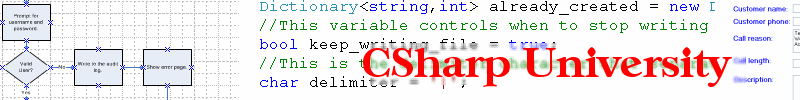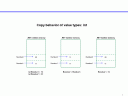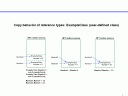"Join my free Amazing ASP.NET newsletter"
It's safe and easy to signup. You get:
• Notified anytime a new article comes out
• Technical step-by-step tutorials on all important ASP.NET topics
• Expert guidance so you can reach unlimited ASP.NET success

In this lesson, I will cover what the difference is between C# value variables (types) and C# reference variables (types).  It is especially important that you understand how reference types work because if you master how to use them properly, you can improve your programming skills and become good at Object-Oriented programming.

Let me first say that if you find this lesson confusing or don’t understand everything at first, go back and read it again either right away or after a short break.  I find that most students new to Object-Oriented programming and C#, find this particular lesson and the material a bit puzzling at first.  If that is you, don’t worry because you are not alone and you will do fine once you practice the concepts taught in here a little bit on your own.

In C#, you can think of Value variables (sometimes called value types) as the built-in types that the language offers.  These are int, bool, decimal, long, etc.  These types of variables directly contain a value once you perform an assignment operation.  Here is an example of a Value variable declaration and assignment.

```int Number1 = 11;
```

Reference variables (sometimes called reference types) are a little bit different.  They are not built-in simple types, but are either .NET Framework defined or User defined classes.  Reference variables are essentially pointers to objects that were created in the .NET runtime memory.  Reference variables do NOT directly contain a value, they are merely pointers to objects that can contain values.  Here is an example of a Reference variable declaration and assignment for a user-defined class called ExampleClass.

```ExampleClass Number1 = new ExampleClass();
Number1.Number = 11;
```

Notice that the “new” keyword is used for the Reference variable declaration.  This is required with Reference variables.  The C# “new” keyword tells .NET to allocate enough memory to hold an ExampleClass object and then the assignment operator returns a pointer (or reference) to the new memory location and it gets placed in the variable Number1.

Let’s explore this a bit further with a more in depth example.  A technique that I like to use in order to demonstrate how Value types are different from Reference types is to show the behavior of the copy operation (assignment operator).  When you assign one Value variable to another Value variable, data is actually copied from one variable to the other.  The original source variable value is left untouched.  I created a diagram to demonstrate this (click to enlarge).In the example diagram, two Value variables are declared.  Then Number2 is assigned to Number1, so Number2 gets a copy of the value in Number1.  Therefore if Number2 is modified to 33, Number1 remains unchanged.

With Reference variables, if you assign one to another, nothing is copied, but instead the destination variable simply gets a pointer (or reference) to the object that the source variable points to.  See the demonstration diagram.In the example diagram, two Reference variables are declared.  Then Number2 is assigned to Number1, but Number2 does NOT get a copy of Number1.  Number2 gets a reference to whatever Number1 is pointing to.  When Number2 is modified, it affects Number1 also, because they are both referencing the SAME ExampleClass object in memory.  I usually see some funny faces on my students in the classroom right about now.  When Number2 is assigned a value of 33, the number that Number1 is referencing is also changed since it is the same object.  Let’s explore these concepts with some more code that matches the diagrams above.

I created an example method to demonstrate the C# behavior of Value variables.

```int Number1 = 11;
int Number2 = 22;

//A copy of the value in Number1 is put in Number2
//during the assignment operation.
Number2 = Number1;
//The value in Number2 is changed.
Number2 = 33;
```

Even though Number2 was modified to 33, it did NOT affect the value of Number1 and you can see that if you run the example code files that are part of this lesson.

Now with Reference variables it is a bit different.

```ExampleClass Number1 = new ExampleClass();
ExampleClass Number2 = new ExampleClass();
//The variables are initialized by putting values
//after the assignment operator.
Number1.Number = 11;
Number2.Number = 22;

//A reference (pointer) to Number1 is put in Number2
//during the assignment operation.
Number2 = Number1;
//Now both variables Number1 and Number2 both reference
//or point to the same object.

//The value in Number2 is changed.  Since both variables
//reference the same object, Number1 is modified as well.
Number2.Number = 33;
```

When Number2 was modified, it also modified Number1 since after the assignment operation, they both reference the same ExampleClass object.  If you run the example code files, you will see that Number1 is also 33 after the line Number2.Number = 33.  Go back and look at the diagram above again.  You will notice that originally Number2 pointed to its own ExampleClass object and there was a separate place in memory for that.  However, after Number1 was assigned to Number2, the memory space that Number2 was originally pointing to is no longer there and Number2 points to the same place that Number1 points to.  The object that Number2 was originally pointing to is no longer being referenced and it is essentially gone forever.  This important to understand because it will help you understand the behavior of C# programs, especially when objects that are not built-in types are being used.

Let’s talk for a second about common places where you have to pay special attention to reference variables: loops.  If you declare a reference type in a loop in C# and each time you use the “new” keyword in each loop iteration, you are creating a new place in memory each time the loop iterates and any references to the old objects in memory are lost.  Take a look at the following code.

```for(int i=1; i<=2; i++)
{
ExampleClass myclass = new ExampleClass();
myclass.Number = i;
}
```

In the loop, every time it iterates, a brand new ExampleClass object will be created and any reference to the object created in the prior iteration of the loop is lost.  Now why am I covering this you may ask, since it is similar to how Value variables work in a loop?  Well I want you to understand that using Reference variables in a loop with the “new” keyword will create a new place in memory every time and there is a way to keep all the references to each new ExampleClass object every time it is created.  The way to do this is by using an Array or other list type to store all of the references created in the loop.  Take a look at this.

```ExampleClass[] classarray = new ExampleClass;
for (int i = 1; i <= 2; i++)
{
ExampleClass myclass = new ExampleClass();
myclass.Number = i;
classarray[i - 1] = myclass;
}
```

I declared an array of type ExampleClass.  Then during each iteration of the loop, I saved a reference to the ExampleClass object being created into the array.  After the loop finishes, I still have a reference to all of the ExampleClass objects that were created.  This technique of storing the pointers (or references) to objects can be used to help make your programs more Object-Oriented since you will be encouraged to create more User-defined classes.

One last thing I want to cover before I closeout this lesson is regarding the “string” variable type.  Technically, Microsoft defines string variables as Reference types.  However their behavior is similar to Value types when the assignment operator is used.  When you assign one string variable to another string variable, it creates a new memory space for the destination variable and the value from the source is copied.  This is in contrast to what we witnessed with other Reference types in this lesson.

I recommend that after downloading the source code for this lesson that you play around and experiment with your own Value and Reference variables to reinforce what we covered here.  Go back to the diagrams if necessary to help you picture what is going on in the C# code.